Get instant live expert help with Excel or Google Sheets“My Excelchat expert helped me in less than 20 minutes, saving me what would have been 5 hours of work!”

#### Post your problem and you’ll get Expert help in seconds.

Your message must be at least 40 characters
Our professional Expert are available now. Your privacy is guaranteed.

# How to Lookup with Variable Sheet Name

We can apply the VLOOKUP and INDIRECT functions to look up data in a variable sheet name in Excel. With the following illustrations, we will learn how to use these functions in simple steps.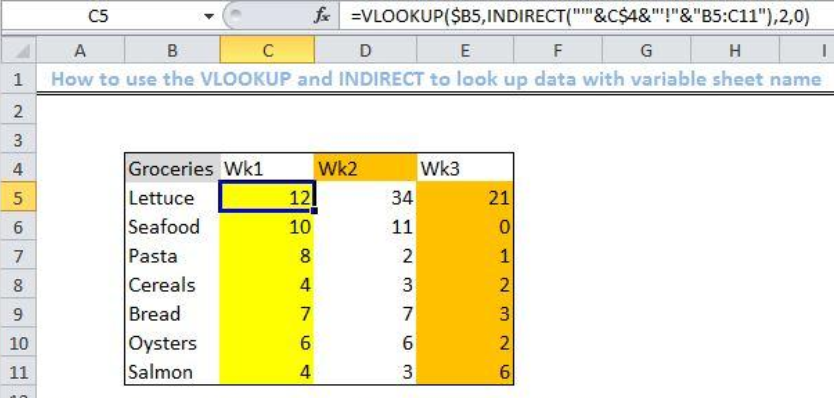Figure 1- Results of using the Excel VLOOKUP and INDIRECT function

## General Formula

`=VLOOKUP(val,INDIRECT("'"&sheet&"'!"&"range"),col,0)`

## Formula

`=VLOOKUP(\$B5,INDIRECT("'"&C\$4&"'!"&"B5:C11"),2,0)`

## Setting up the Data

• We will assign a name to the blank sheet called “Wk1.
• In this sheet, we will set up our hypothetical data titled “Groceries” and “Quantity” in Columns B and C as displayed in the figure below.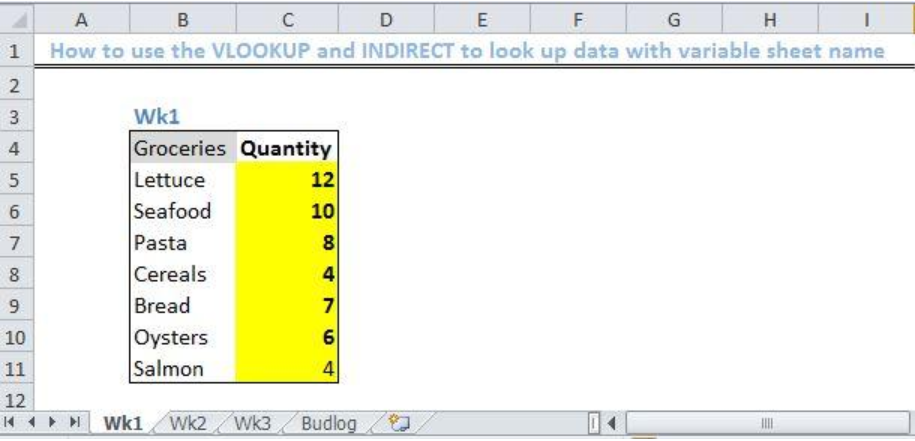Figure 2 – Setting up Datasheet”Wk1.”

• We will create identical sheets with different values for the quantity section as found in the “Wk1” in sheets Wk2, and Wk3 respectively.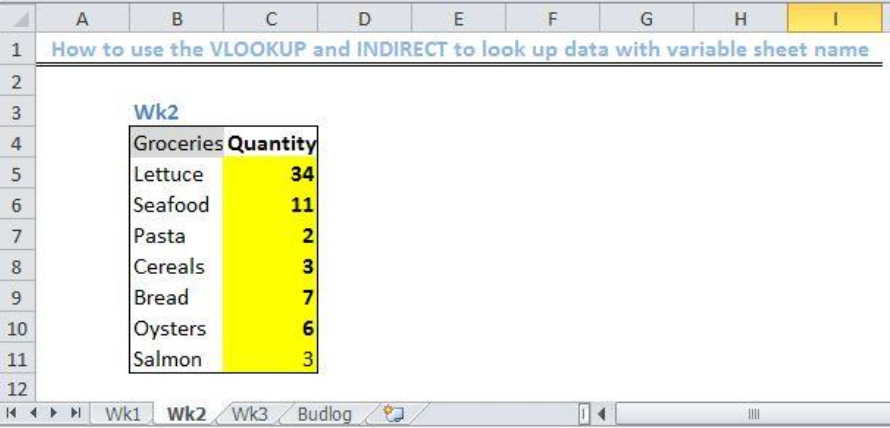Figure 3- Setting up Datasheet “Wk2.”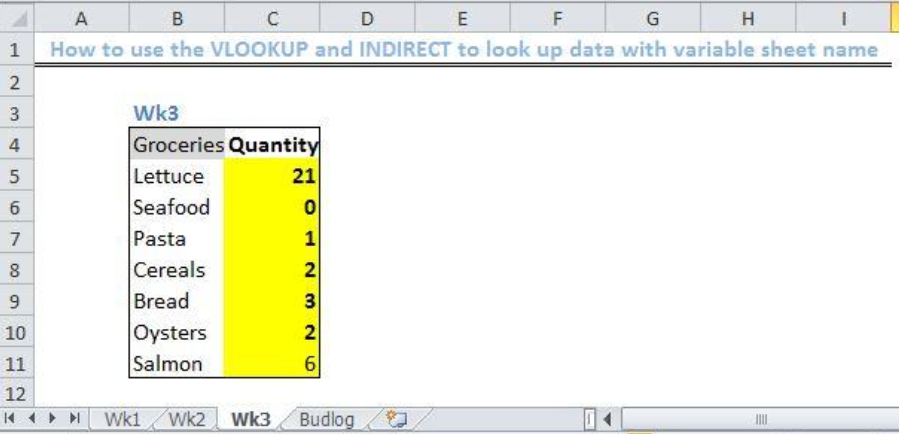Figure 4 – Setting up Datasheet “Wk3.”

• We will create a 4th sheet, titled “Budlog.” Here we will summarize our data.
• In this sheet, we will enter the following names in Cells C4 to E4, “wk1”, “wk2”, and “wk3.
• We will set up our hypothetical list of data titled Groceries in Columns B.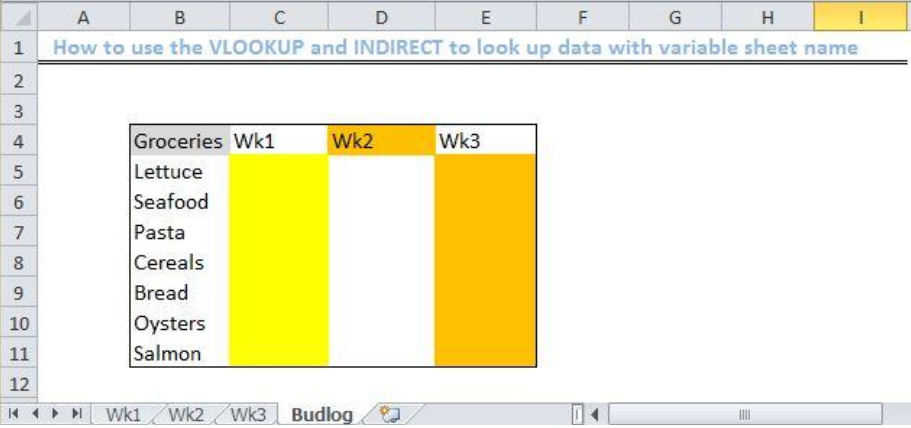Figure 5- Setting up Data Sheet “Budlog”

• To lookup data, we will pick a cell in the sheet: budlog”, assign the VLOOKUP formula and extract the data by creating a dynamic reference to the sheet name for the Budget sheet.

## Applying the VLOOKUP and INDIRECT function

• We select the Cell C5
• We will enter the formula below on Cell C5
`=VLOOKUP(\$B5,INDIRECT("'"&C\$4&"'!"&"B5:C11"),2,0)`
• We will press the enter key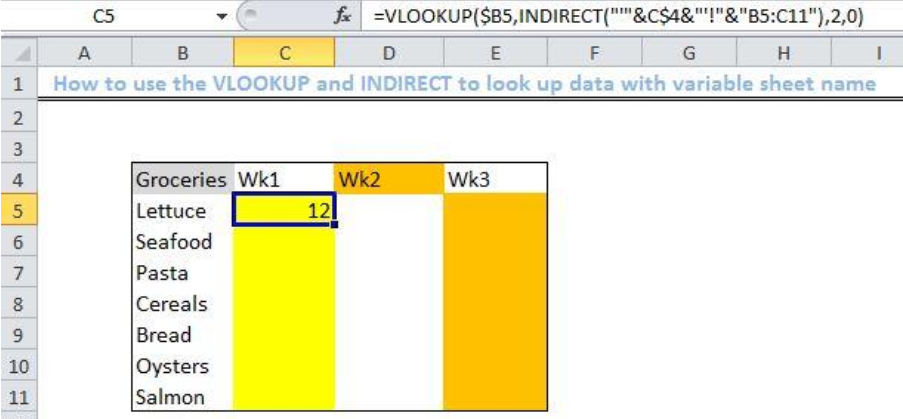Figure 6- Applying the VLOOKUP and INDIRECT functions

• We select Cell C5 again
• Next, we use the fill handle tool (the small plus sign found at the bottom of Cell C5) to drag the formula from Cell C5 to Cell E11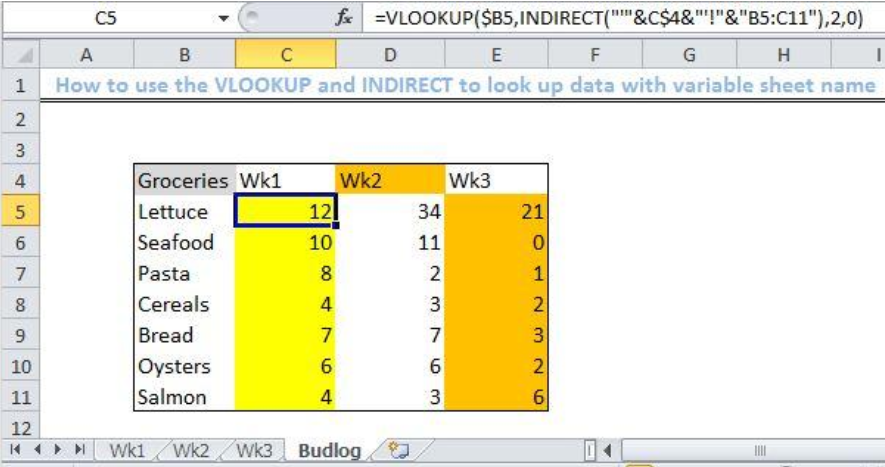Figure 7- Result of using the VLOOKUP and INDIRECT functions

## Explanation

`=VLOOKUP(\$B5,INDIRECT("'"&C\$4&"'!"&"B5:C11"),2,0)`

The VLOOKUP function will check all data inside the INDIRECT function. It will use the dynamic reference to name the sheets prepared in the Excel workspace. The mixed reference is represented by \$B5 which will lock Column B and allow for copying across the table.

`=INDIRECT("'"&C\$4&"'!B5:C11")`

The INDIRECT function in this situation will refer to the column headings in row 4 and match it against the sheet names in the workbook (i.e., “wk1”, “wk2”, “wk3”)

## Note

• If the VLOOKUP function does not find a match with our lookup value, it will return with a #N/A error.

## Instant Connection to an Expert through our Excelchat Service

Most of the time, the problem you will need to solve will be more complex than a simple application of a formula or function. If you want to save hours of research and frustration, try our live Excelchat service! Our Excel Experts are available 24/7 to answer any Excel question you may have. We guarantee a connection within 30 seconds and a customized solution within 20 minutes.

### Did this post not answer your question? Get a solution from connecting with the expert.Another blog reader asked this question today on Excelchat:
Solution examplesHi I'm trying to use fuzzy lookup. Not sure how it's working. I have two strings which are different ('Unemployment Benefits' vs 'Invalid pensioner'), yet getting a similarity index of 0.9474.
Solved by E. E. in 40 minsGood Morning, I am trying to get parts from one column in an excel spread sheet to match up with a parts and cost column from a different excel spread sheet. (Not all parts on each spread sheet are the same)
Solved by S. L. in 49 minsI am trying to perform a vlookup or index match for a large data set. Normally I rely on vlookup but it is giving me data from the wrong column whatever I do. I have tried to move to vlookup and it is doing exactly the same thing. Do you have any tips?
Solved by O. W. in 27 minsI need help with Vlookup- am trying to copy a data from one sheet onto another matching the number but with a column
Solved by C. H. in 20 minsI need to formulate a cell where it will show different values. for example if d2=0 i need e2 to show N. But the range of d2 could be anywhere from 0-60
Solved by I. U. in 29 mins## Subscribe to Excelchat.coAnother blog reader asked this question today on Excelchat: